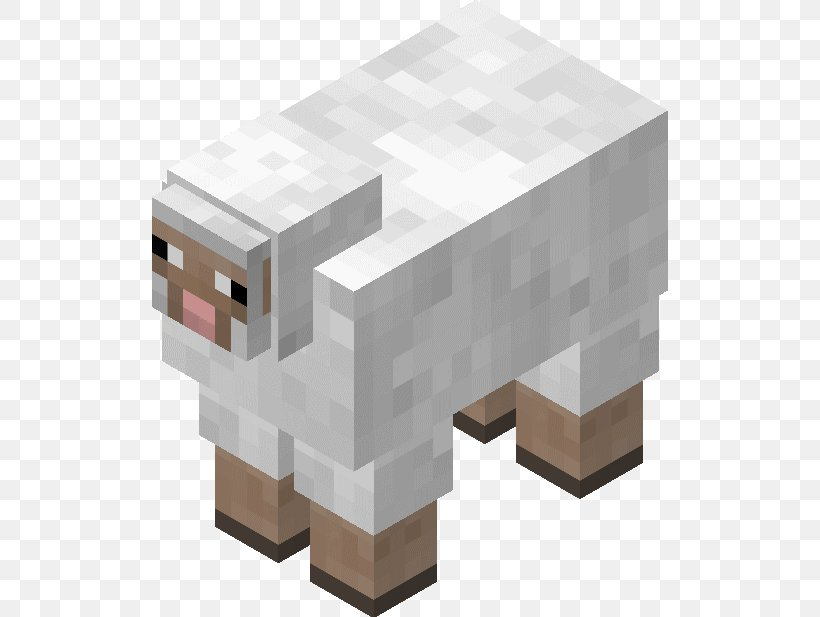Learn to Code via Tutorials on Repl.it!

← Back to all posts
How (I assume) Minecraft mobs move around!

# This is how I assume Minecraft mobs move around like sheep!Lets say there is a sheep. It moves around randomly, right? Right. But how does it move around? This is a lesson on how (I assume) it moves around.

# Step 1: Directions!

Lets start with directions. There are 4 directions. Top, down,(Front and back for 3D, but for now, were keeping it 2D) Left and right! Keep this in mind for later!

# Step 2: Random stuff!

And now we will be focusing a bit on `random`. We all know that computers can pick random stuff right? Well, for those of you who somehow don't know, they can. And now we can make the mobs move around! Not yet. But were almost there! And now we have our first tiny bit of code!

``````//JavaScript
function randint(min, max) {
return Math.floor(Math.random() * (max - min)) + min;
}
var rand = randint(1, 10);

All we need is 2 more things!

# Step 3: Switch statements!

What is `switch`? The `switch` is a statement that is basically a bunch of `if`, `else if`, and `else` stuff made a LOT less messier. For those of you who only know Python, than you might not understand this stuff. Anyways, this stuff will help us a bit in this. It SHOULD have worked for me, but for some reason it did not for reasons that are a bit too complicated. So were going to keep it with good old if, else if, and else. And we now have more code!

``````//JavaScript
//Some chunk of code to make random numbers easier to do, you dont have to do this
function randint(min, max) {
return Math.floor(Math.random() * (max - min)) + min;
}
var x = 0;
var y = 0;
var direction = randint(1, 5);
//Should have been switch statement! But did not work!
if (direction == 1) {
x = x + 25;
}
else if (direction == 2) {
x = x - 25;
}
else if (direction == 3) {
y = y + 25;
}
else if (direction == 4) {
y = y - 25;
}``````

Learn more about switch for JS Here!. Its the same thing for C++ but Im going to link it for C++ Here!. Its also a same for a few other languages.

# Step 4, applying it to some pictures!

Now all we have to do is apply it to some pictures! Here it is in HTML:

``````<!--HTML-->
<img src="black_image.jpg" alt="pixel" id="Pixel" width="25px" height="25px" onclick="poop()"> ``````

In JavaScript (Its actually ALL the code from the js file):

``````//JavaScript
function randint(min, max) {
return Math.floor(Math.random() * (max - min)) + min;
}
var x = 0;
var y = 0;
//setInterval is like a loop, but its timed every time it starts again.
setInterval(
//Was supposed to be switch statement.
function () {
var direction = randint(1, 5);
if (direction == 1) {
x = x + 25;
}
else if (direction == 2) {
x = x - 25;
}
else if (direction == 3) {
y = y + 25;
}
else if (direction == 4) {
y = y - 25;
}

//Set boundries, you dont need it in minecraft since its infinite!
if (x <= 0) {
x = x + 25;
}
else if (x >= 1000) {
x = x - 25;
}
else if (y <= 0) {
y = y + 25;
}
else if (y >= 1000) {
y = y - 25;
}

var el = document.getElementById("Pixel");
el.style.position = "absolute";
el.style.top = y + "px";
el.style.left = x + "px";
document.getElementById("Direction").innerHTML = "direction: " + direction;
}, 1000
)``````

I will make some tutorials for the images. In the meantime, here are some other tutorials:
W3schools
MDN web docs
TutorialsPoint

# What does this have to do with Minecraft?

This is just a really-simple version of what Minecraft mobs do. Except they are WAY more advanced because if a hostile mob detects a player or if a you are holding wheat and sheep are nearby. And flying mobs are WAY harder to code I guess.

# The end!

I hope you enjoyed this tutorial and PLEASE upvote it if you learned something new! And check out the repl below to see it all in action! And thanks to @Coder100 for helping me with the images moving around!

I dont know how to conclude this so bye!